# vs.eyeandcontacts.com

## Chapter 13 Exponents and Powers Exercise 13.2

Question 1: Using laws of exponents, simplify and write the answer in exponential form:
(i) 3² × 3⁴ × 3⁸(ii) 6¹⁵ ÷ 6¹⁰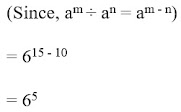(iii) a³ × a²(iv) 7x × 7²(v) (5²)³ ÷ 5³(vi) 2⁵ × 5(vii) a⁴ × b(viii) (3⁴)³(ix) (2²⁰ ÷ 2¹⁵) × 2³(x) 8t ÷ 8²Question 2: Simplify and express each of the following in exponential form:
(i) 2³ × 3
⁴ × 4/3 × 32(ii) ((5²)³ × 5⁴) ÷ 5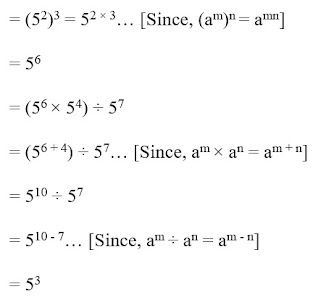(iii) 25⁴ ÷ 5³(iv) 3 × 7² × 11⁸/21 × 11³(v) 3⁷/3⁴ × 3³(vi) 2⁰ + 3⁰ + 4(vii) 2⁰ × 3⁰ × 4(viii) (3⁰ + 2⁰) × 5(ix) 2⁸ × a⁵/4³ × a³(x) a⁵/a³ × a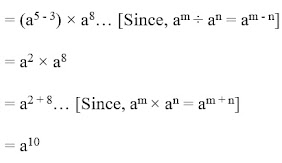(xi) 4⁵ × a⁸b³/4⁵ × a⁵b²(xii) (2³ × 2)²Question 3: Say true or false and justify your answer:
(i) 10 × 10
¹¹ = 100¹¹(ii) 2³ > 5²(iii) 2³ × 3² = 6(iv) 3⁰ = (1000)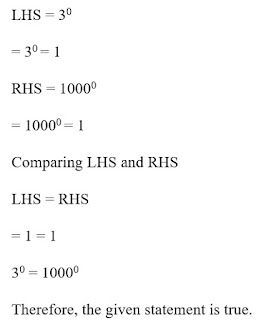Question 4: Express each of the following as a product of prime factors only in exponential form:
(i) 108 × 192(ii) 270(iii) 729 × 64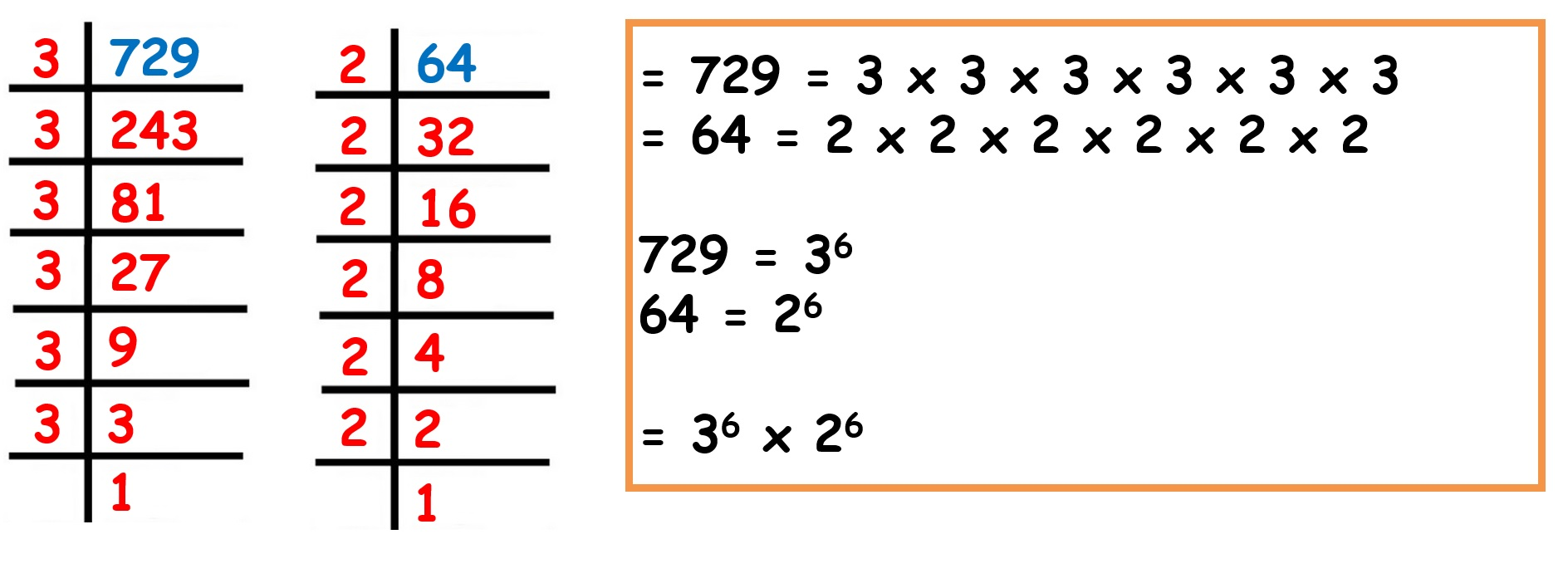(iv) 768Question 5: Simplify:
(i) (2
⁵)² × 7³/8³ × 7(ii) 25 × 5² × t⁸/10³ × t